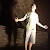##### Co.Create Club
Join Co.Create Club
••••••••const bar = (num) => {

if (num < 2) {

return 1;

}

return num * bar(num-1);

}

How i can solved this? there are two side provide in question : input number and expected result. From this data, we can analize => this function has a stopper and pattern.

x   |   pattern           | expected result      | stopper ?

0 –>                      = 1 (because 0 < 2)  —> this is stopper

1 –> 1                      = 1 (because 1 < 2)  —> this is stopper

2 –> 2 * 1                = 2

3 –> 3 * 2 * 1           = 6

5 –> 5 * 4 * 3 * 2 * 1 = 120

so, the general pattern :

x >= 2  ::::  x * (x-1) * (x-2) * …. * 1
x < 2    ::::  1

Thank you.

29 August, 2019 Like ( 0 )
###### Share